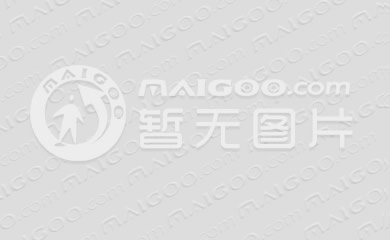# 钢木门生意怎么样 钢木门加盟费是多少• 钢木门是一种结构为钢板与木材相混合的门，它的许多制作工艺都是从防盗门转移过来的，但是由于材料更坚实，安全性、防盗性比其他门都更加强。钢木门由于制作工艺精良、性能优良，价格适中，受到了许多消费者的青睐和追捧。目前随着人们生活水平的提高和对家庭防盗问题的重视，越来越多的家庭会选择安装使用钢木门。钢木门不仅在一二线城市受欢迎，在三四线城市的销售情况也挺好。所以做钢木门生意是一个不错的选择。

 加盟店型 省会城市 地级城市 县级城市 加盟店面积 80平米 80平米 80平米 装修费 5.6万元（700元/㎡） 4.8万元（600元/㎡） 4万元（500元/㎡） 经营设备费 4万元 3.5万元 3万元 首批进货费 7万元 6万元 5万元 宣传推广费用 6000元 5000元 4000元 店面租金 0.85万元/月 0.7万元/月 0.55万元/月 店员工资 12000元（4000元/人*3） 10800元（3600元/人*3） 9600元（3200元/人*3） 水电杂费 1200元 1100元 1000元 流动备用资金 10万元 8万元 6万元 加盟投资总费用 19.37万元 16.69万元 14.01万元

0*您的称呼
*代理区域
*手机号码
*意向行业
• 集成灶具
• 顶墙整装
• 地板材料
• 墙布墙纸
• 管道水管
• 净水器设备
• 全屋定制家居
• 艺术漆
• 油漆涂料
• 板材木材
• 门窗木门
• 智能锁门锁
• 学习桌椅
• 定制橱柜
• 瓷砖陶瓷
• 卫浴洁具
• 暖气片供暖
• 胶水美缝剂
• 古典红木家具
• 集成灶
• 集成水槽
• 燃气灶
• 油烟机
• 消毒柜
• 蒸箱
• 洗碗机
• 烤箱
• 集成吊顶
• 集成墙面
• 铝扣板
• 轻钢龙骨
• 浴霸
• 全屋整装
• 铝单板
• 地板
• 强化地板
• 实木地板
• 多层地板
• 竹地板
• 防静电地板
• 地热地板
• 塑胶地板
• 橡胶地板
• 软木地板
• 木塑地板
• 网络地板
• 弹性地板
• 运动地板
• 石塑地板
• 墙布
• 墙纸
• 水管管道
• 进口水管
• 穿线管
• 地暖管
• pp管
• ppr管
• pe管
• pvc管
• 净水器
• 纯水机
• 直饮机
• 饮水机
• 软水机
• 净水龙头
• 中央净水器
• 全屋定制
• 整体衣柜
• 整木家装
• 壁柜
• 衣柜门
• 榻榻米
• 玄关柜
• 板式家具
• 硅藻泥
• 艺术漆/艺术涂料
• 贝壳粉
• 腻子粉
• 油漆
• 乳胶漆
• 水性漆
• 木器漆
• 防火涂料
• 防水涂料
• 防腐涂料
• 建筑涂料
• 工业油漆
• 真石漆
• 粉末涂料
• 仿石涂料
• 氟碳漆
• 板材
• 生态板
• 细木工板
• 胶合板
• 饰面板
• 密度板
• 刨花板
• 集成材
• 防腐木
• 防火板
• 阻燃板
• 护墙板
• 吸音板
• 阳光板
• 装饰纸
• 人造板
• 碳化木
• 实木门
• 推拉门
• 防盗门
• 木门
• 室内门
• 免漆门
• 钢木门
• 防火门
• 玻璃门
• 静音门
• 生态门
• 时尚门
• 非标门
• 木塑门
• 楼宇门
• 铜门
• 折叠门
• 自动门
• 全屋门窗
• 铝合金门窗
• 不锈钢门窗
• 铝包木门窗
• 塑钢门窗
• 系统门窗
• 卷帘门窗
• 防盗窗
• 纱窗
• 隔音窗
• 防火窗
• 家装建材
• 门锁
• 智能锁/指纹锁
• 密码锁
• 防盗门锁芯
• 执手锁
• 酒店锁
• 挂锁
• 玻璃门锁
• 学习桌
• 书桌
• 电脑桌
• 桌子
• 儿童椅
• 课桌椅
• 电脑椅
• 办公桌
• 橱柜
• 不锈钢橱柜
• 整体厨房
• 瓷砖/地板砖
• 内墙砖
• 外墙砖
• 抛光砖
• 釉面砖
• 大理石瓷砖
• 木纹砖
• 玻化砖
• 马赛克瓷砖
• 通体砖
• 亚光砖
• 微晶石
• 仿古砖
• 大理石
• 石材石料
• 日用陶瓷
• 洁具卫浴
• 花洒
• 水龙头
• 马桶
• 智能马桶
• 台盆
• 浴室柜
• 浴缸
• 按摩浴缸
• 淋浴房
• 蹲便器
• 感应洁具
• 地漏
• 整体卫浴
• 小便斗
• 角阀
• 卫浴五金
• 全卫定制
• 水槽
• 冲水箱
• 马桶盖
• 暖气片
• 采暖器
• 供暖
• 美缝剂
• 瓷砖胶
• 石材胶
• 防水胶
• 结构胶
• 发泡胶
• 界面剂
• 玻璃胶
• 腻子
• 红木家具
• 古典家具
• 实木家具
• 榆木家具
• 分体式集成灶
• 高端建材
• 外国建材
• 家居建材
• 灯具建材
• 新型建材
• 五金建材
• 建材市场
*您的留言